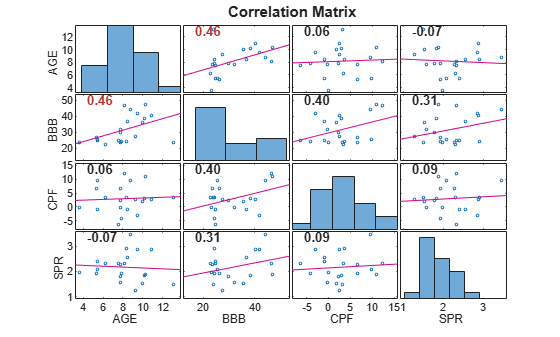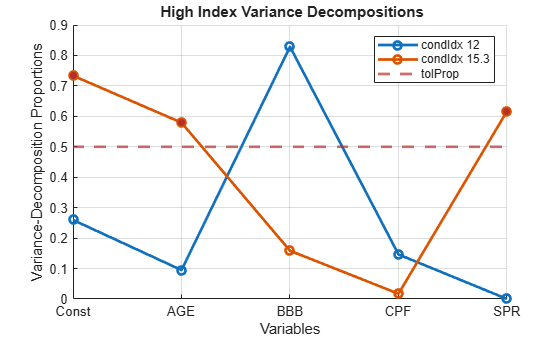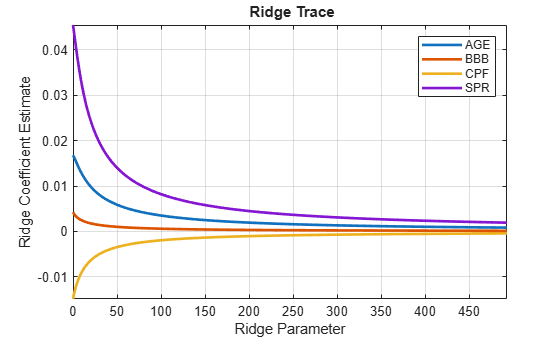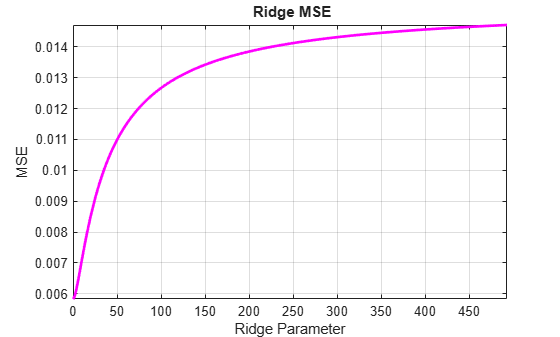# Time Series Regression II: Collinearity and Estimator Variance

This example shows how to detect correlation among predictors and accommodate problems of large estimator variance. It is the second in a series of examples on time series regression, following the presentation in the previous example.

### Introduction

Economic models are always underspecified with respect to the true data-generating process (DGP). Model predictors never fully represent the totality of causal factors producing an economic response. Omitted variables, however, continue to exert their influence through the innovations process, forcing model coefficients to account for variations in the response that they do not truly explain. Coefficient estimates that are too large (type I errors) or too small (type II errors) distort the marginal contribution of each predictor. In some cases, coefficients even end up with the wrong sign.

Economic models can also be overspecified, by including a theory-blind mix of predictors with the hope of capturing some significant part of the DGP. Often, "general-to-specific" (GETS) estimation methods are applied with a misplaced trust that standard diagnostic statistics will sort out the good predictors. However, the very presence of causally insignificant predictors tends to increase estimator variance, raising the possibility that standard inferences will be unreliable.

The reality of working with misspecified models is addressed in this, and subsequent, examples in this series. Underspecified models often introduce correlation between predictors and omitted variables in the innovations process. Overspecified models often introduce correlation among predictors. Each presents its own problems for model estimation. In this example, we look specifically at problems arising from correlated predictors. The somewhat more complicated issues related to correlation between predictors and innovations (exogeneity violations) are addressed in the example Time Series Regression VIII: Lagged Variables and Estimator Bias.

We begin by loading relevant data from the previous example Time Series Regression I: Linear Models, and continue the analysis of the credit default model presented there:

load Data_TSReg1

### Correlation and Condition Numbers

As a first step toward model specification, it is useful to identify any possible dependencies among the predictors. The correlation matrix is a standard measure of the strength of pairwise linear relationships:

R0 = corrcoef(X0)
R0 = 4×4 1.0000 0.4578 0.0566 -0.0686 0.4578 1.0000 0.3955 0.3082 0.0566 0.3955 1.0000 0.0874 -0.0686 0.3082 0.0874 1.0000 

The utility function corrplot helps to visualize the results in the correlation matrix by plotting a matrix of pairwise scatters. Slopes of the displayed least-squares lines are equal to the displayed correlation coefficients. It is convenient to work with the tabular array version of the data, X0Tbl, which contains the predictor names for the plots:

figure corrplot(X0Tbl,'testR','on')Correlation coefficients highlighted in red have a significant $t$-statistic. The predictor BBB again distinguishes itself by its relatively high correlations with the other predictors, though the strength of the relationships is moderate. Here the visualization is particularly helpful, as BBB displays fairly disorganized scatters, with the possibility of a number of small, potentially influential data subsets. The plots are a reminder of the limitations of the linear correlation coefficient as a summary statistic.

Both the scale and correlations of BBB have the potential to inflate the condition number $\kappa$ of ${X}_{t}$. The condition number is often used to characterize the overall sensitivity of OLS estimates to changes in the data. For an MLR model with intercept:

kappa0I = cond(X0I)
kappa0I = 205.8085 

The condition number is well above the "well-conditioned" benchmark of 1, which is achieved when ${X}_{t}$ has orthonormal columns. As a rule of thumb, a 1% relative error in the data ${X}_{t}$ can produce up to a $\kappa$% relative error in the coefficient estimates $\beta$ :

$\frac{‖\delta \beta ‖}{‖\beta ‖}\le \kappa \frac{‖\delta {X}_{t}‖}{‖{X}_{t}‖}$

As shown in the previous example Time Series Regression I: Linear Models, coefficient estimates for this data are on the order of $1{0}^{-2}$, so a $\kappa$ on the order of $1{0}^{2}$ leads to absolute estimation errors $‖\delta \beta ‖$ that are approximated by the relative errors in the data.

### Estimator Variance

Correlations and condition numbers are widely used to flag potential data problems, but their diagnostic value is limited. Correlations consider only pairwise dependencies between predictors, while condition numbers consider only ${X}_{t}$ in aggregate. Relationships among arbitrary predictor subsets (multicollinearities) can fall somewhere in between. CLM assumptions forbid exact relationships, but identifying the strength and source of any near relationships, and their specific effect on coefficient estimation, is an essential part of specification analysis.

Many methods for detecting near collinearities focus on the coefficient estimates in $\underset{}{\overset{ˆ}{\beta }}$, rather than the data in ${X}_{t}$. Each of the following has been suggested as a telltale sign of predictor dependencies:

• Statistically insignificant coefficients on theoretically important predictors

• Coefficients with signs or magnitudes that do not make theoretical sense

• Extreme sensitivity of a coefficient to insertion or deletion of other predictors

The qualitative nature of these criteria is apparent, and unfortunately none of them is necessary or sufficient for detecting collinearity.

To illustrate, we again display OLS fit statistics of the credit default model:

M0
M0 = Linear regression model: IGD ~ 1 + AGE + BBB + CPF + SPR Estimated Coefficients: Estimate SE tStat pValue _________ _________ _______ _________ (Intercept) -0.22741 0.098565 -2.3072 0.034747 AGE 0.016781 0.0091845 1.8271 0.086402 BBB 0.0042728 0.0026757 1.5969 0.12985 CPF -0.014888 0.0038077 -3.91 0.0012473 SPR 0.045488 0.033996 1.338 0.1996 Number of observations: 21, Error degrees of freedom: 16 Root Mean Squared Error: 0.0763 R-squared: 0.621, Adjusted R-Squared: 0.526 F-statistic vs. constant model: 6.56, p-value = 0.00253 

The signs of the coefficient estimates are consistent with theoretical expectations: AGE, BBB, and SPR add risk; CPF reduces it. The t-statistics, which scale the coefficient estimates by their standard errors (computed under the assumption of normal innovations), show that all predictors are significantly different from 0 at the 20% level. CPF appears especially significant here. The significance of a predictor, however, is relative to the other predictors in the model.

There is nothing in the standard regression results to raise substantial concern about collinearity. To put the results in perspective, however, it is necessary to consider other sources of estimator variance. Under CLM assumptions, the variance of the ${i}^{th}$ component of $\underset{}{\overset{ˆ}{\beta }}$, $\underset{}{\overset{ˆ}{{\beta }_{i}}}$, can be decomposed as follows :

$Var\left(\underset{}{\overset{ˆ}{{\beta }_{i}}}\right)=\frac{{\sigma }^{2}}{SS{T}_{i}\left(1-{R}_{i}^{2}\right)},$

where ${\sigma }^{2}$ is the variance of the innovations process (assumed constant), $SS{T}_{i}$ is the total sample variation of predictor $i$, and ${R}_{i}^{2}$ is the coefficient of determination from a regression of predictor $i$ on the remaining predictors (and intercept, if present).

The term

$VI{F}_{i}=\frac{1}{1-{R}_{i}^{2}}$

is called the variance inflation factor (VIF), and is another common collinearity diagnostic. When the variation of predictor $i$ is largely explained by a linear combination of the other predictors, ${R}_{i}^{2}$ is close to $1$, and the VIF for that predictor is correspondingly large. The inflation is measured relative to an ${R}_{i}^{2}$ of 0 (no collinearity), and a VIF of 1.

VIFs are also the diagonal elements of the inverse of the correlation matrix , a convenient result that eliminates the need to set up the various regressions:

VIF = diag(inv(R0))'
VIF = 1×4 1.3870 1.7901 1.2216 1.1850 
predNames0
predNames0 = 1x4 cell {'AGE'} {'BBB'} {'CPF'} {'SPR'} 

How large a VIF is cause for concern? As with significance levels for standard hypothesis tests, experience with certain types of data may suggest useful tolerances. Common ad hoc values, in the range of 5 to 10, are of little use in general. In this case, BBB has the highest VIF, but it does not jump out from the rest of the predictors.

More importantly, VIF is only one factor in the variance decomposition given above. A large VIF can be balanced by either a small innovations variance ${\sigma }^{2}$ (good model fit) or a large sample variation $SS{T}_{i}$ (sufficient data). As such, Goldberger  ironically compares the "problem" of multicollinearity, viewed in isolation, to the problem of data "micronumerosity." Evaluating the combined effect of the different sources of estimator variance requires a wider view.

Econometricians have developed a number of rules of thumb for deciding when to worry about collinearity. Perhaps the most common says that it is acceptable to ignore evidence of collinearity if the resulting t-statistics are all greater than 2 in absolute value. This ensures that 0 is outside of the approximate 95% confidence interval of each estimate (assuming normal innovations or a large sample). Because t-statistics are already adjusted for estimator variance, the presumption is that they adequately account for collinearity in the context of other, balancing effects. The regression results above show that three of the potential predictors in X0 fail this test.

Another rule of thumb is based on an estimate of $Var\left(\underset{}{\overset{ˆ}{{\beta }_{i}}}\right)$ :

$\underset{}{\overset{ˆ}{Var}}\left(\underset{}{\overset{ˆ}{{\beta }_{i}}}\right)=\frac{1}{T-n}\frac{{\underset{}{\overset{ˆ}{\sigma }}}_{y}^{2}}{{\underset{}{\overset{ˆ}{\sigma }}}_{i}^{2}}\frac{1-{R}^{2}}{1-{R}_{i}^{2}},$

where $T$ is the sample size, $n$ is the number of predictors, ${\underset{}{\overset{ˆ}{\sigma }}}_{y}^{2}$ is the estimated variance of ${y}_{t}$, ${\underset{}{\overset{ˆ}{\sigma }}}_{i}^{2}$ is the estimated variance of predictor $i$, ${R}^{2}$ is the coefficient of determination for the regression of ${y}_{t}$ on ${X}_{t}$, and ${R}_{i}^{2}$ is as above. The rule says that concerns about collinearity can be ignored if ${R}^{2}$ exceeds ${R}_{i}^{2}$ for each predictor, since each VIF will be balanced by $1-{R}^{2}$. All of the potential predictors in X0 pass this test:

RSquared = M0.Rsquared
RSquared = struct with fields: Ordinary: 0.6211 Adjusted: 0.5264 
RSquared_i = 1-(1./VIF)
RSquared_i = 1×4 0.2790 0.4414 0.1814 0.1561 
predNames0
predNames0 = 1x4 cell {'AGE'} {'BBB'} {'CPF'} {'SPR'} 

These rules attempt to identify the consequences of collinearity, as expressed in the regression results. As we have seen, they can offer conflicting advice on when, and how much, to worry about the integrity of the coefficient estimates. They do not provide any accounting of the nature of the multiple dependencies within the data, nor do they provide any reliable measure of the extent to which these dependencies degrade the regression.

### Collinearity Diagnostics

A more detailed analytic approach is provided in . Instability of OLS estimates can be traced to small eigenvalues in the cross-product matrix ${X}_{t}^{T}{X}_{t}$ appearing in the normal equations for $\underset{}{\overset{ˆ}{\beta }}$:

$\underset{}{\overset{ˆ}{\beta }}=\left({X}_{t}^{T}{X}_{t}{\right)}^{-1}{X}_{t}^{T}{y}_{t}.$

Belsley reformulates the eigensystem of ${X}_{t}^{T}{X}_{t}$ in terms of the singular values of the matrix ${X}_{t}$, which can then be analyzed directly, with greater numerical accuracy. If the singular values of ${X}_{t}$ are ${\mu }_{1},...,{\mu }_{n}$, where $n$ is the number of predictors, then the condition number of ${X}_{t}$ is $\kappa ={\mu }_{max}/{\mu }_{min}$. Belsley defines a spectrum of condition indices ${\eta }_{j}={\mu }_{max}/{\mu }_{j}$ for each $j=1,...,n$, and shows that high indices indicate separate near dependencies in the data.

Belsley goes further by describing a method for identifying the specific predictors involved in each near dependency, and provides a measure of how important those dependencies are in affecting coefficient estimates. This is achieved with yet another decomposition of $Var\left(\underset{}{\overset{ˆ}{{\beta }_{i}}}\right)$, this time in terms of the singular values. If ${X}_{t}$ has a singular-value decomposition $US{V}^{T}$, with $V=\left({v}_{ij}\right)$, then:

$Var\left(\underset{}{\overset{ˆ}{{\beta }_{i}}}\right)={\sigma }^{2}\sum _{j=1}^{n}{v}_{ij}^{2}/{\mu }_{j}^{2},$

where ${\sigma }^{2}$ is the innovations variance. The variance decomposition proportions ${\pi }_{ji}$ are defined by:

${\varphi }_{ij}={v}_{ij}^{2}/{\mu }_{j}^{2},$

${\varphi }_{i}={\sum }_{j=1}^{n}{\varphi }_{ij},$

${\pi }_{ji}={\varphi }_{ij}/{\varphi }_{i}.$

The ${\pi }_{ji}$ give the proportion of $Var\left(\underset{}{\overset{ˆ}{{\beta }_{i}}}\right)$ associated with singular value ${\mu }_{j}$.

Indices and proportions are interpreted as follows:

• The number of high condition indices identifies the number of near dependencies.

• The size of the condition indices identifies the tightness of each dependency.

• The location of high proportions in a high index row identifies the dependent predictors.

• The size of the proportions identifies the degree of degradation to regression estimates.

Again, a tolerance for "high" must be determined. Belsley's simulation experiments suggest that condition indices in the range of 5 to 10 reflect weak dependencies, and those in the range 30 to 100 reflect moderate to high dependencies. He suggests a tolerance of 0.5 for variance decomposition proportions identifying individual predictors. Simulation experiments, however, are necessarily based on specific models of mutual dependence, so tolerances need to be reevaluated in each empirical setting.

The function collintest implements Belsley's procedure. Outputs are displayed in tabular form:

collintest(X0ITbl);
Variance Decomposition sValue condIdx Const AGE BBB CPF SPR --------------------------------------------------------- 2.0605 1 0.0015 0.0024 0.0020 0.0140 0.0025 0.8008 2.5730 0.0016 0.0025 0.0004 0.8220 0.0023 0.2563 8.0400 0.0037 0.3208 0.0105 0.0004 0.3781 0.1710 12.0464 0.2596 0.0950 0.8287 0.1463 0.0001 0.1343 15.3405 0.7335 0.5793 0.1585 0.0173 0.6170 

If we lower the index tolerance to 10 and maintain a proportion tolerance of 0.5, the analysis identifies one weak dependency between AGE and SPR in the final row. It can be visualized by setting the 'tolIdx' and 'tolProp' parameters in collintest and turning on the 'plot' flag:

figure collintest(X0ITbl,'tolIdx',10,'tolProp',0.5,'display','off','plot','on');The plot shows critical rows in the variance decomposition table, above the index tolerance. The row associated with condition index 12 has only one predictor, BBB, with a proportion above the tolerance, not the two or more predictors required for a dependency. The row associated with condition index 15.3 shows the weak dependence involving AGE, SPR, and the intercept. This relationship was not apparent in the initial plot of the correlation matrix.

In summary, the results of the various collinearity diagnostics are consistent with data in which no degrading near relationships exist. Indeed, a review of the economic meaning of the potential predictors (easily lost in a purely statistical analysis) does not suggest any theoretical reason for strong relationships. Regardless of weak dependencies, OLS estimates remain BLUE, and the standard errors in the regression results show an accuracy that is probably acceptable for most modeling purposes.

### Ridge Regression

To conclude, we briefly examine the technique of ridge regression, which is often suggested as a remedy for estimator variance in MLR models of data with some degree of collinearity. The technique can also be used as a collinearity diagnostic.

To address the problem of near singularity in ${X}_{t}^{T}{X}_{t}$, ridge regression estimates $\underset{}{\overset{ˆ}{\beta }}$ using a regularization of the normal equations:

${\underset{}{\overset{ˆ}{\beta }}}_{ridge}=\left({X}_{t}^{T}{X}_{t}+kI{\right)}^{-1}{X}_{t}^{T}{y}_{t},$

where $k$ is a positive ridge parameter and $I$ is the identity matrix. The perturbation to the diagonal of ${X}_{t}^{T}{X}_{t}$ is intended to improve the conditioning of the eigenvalue problem and reduce the variance of the coefficient estimates. As $k$ increases, ridge estimates become biased toward zero, but a reduced variance can result in a smaller mean-squared error (MSE) relative to comparable OLS estimates, especially in the presence of collinearity.

Ridge regression is carried out by the function ridge. To examine the results for a range of ridge parameters $k$, a ridge trace  is produced:

Mu0I = mean(diag(X0I'*X0I)); % Scale of cross-product diagonal k = 0:Mu0I/10; % Range of ridge parameters ridgeBetas = ridge(y0,X0,k,0); % Coefficients for MLR model with intercept figure plot(k,ridgeBetas(2:end,:),'LineWidth',2) xlim([0 Mu0I/10]) legend(predNames0) xlabel('Ridge Parameter') ylabel('Ridge Coefficient Estimate') title('{\bf Ridge Trace}') axis tight grid onThe OLS estimates, with $k=0$, appear on the left. The important question is whether any of the ridge estimates reduce the MSE:

[numRidgeParams,numRidgeBetas] = size(ridgeBetas); y0Hat = X0I*ridgeBetas; RidgeRes = repmat(y0,1,numRidgeBetas)-y0Hat; RidgeSSE = RidgeRes'*RidgeRes; RidgeDFE = T0-numRidgeParams; RidgeMSE = diag(RidgeSSE/RidgeDFE); figure plot(k,RidgeMSE,'m','LineWidth',2) xlim([0 Mu0I/10]) xlabel('Ridge Parameter') ylabel('MSE') title('{\bf Ridge MSE}') axis tight grid onThe plot shows exactly the opposite of what one would hope for when applying ridge regression. The MSE actually increases over the entire range of ridge parameters, suggesting again that there is no significant collinearity in the data for ridge regression to correct.

A technique related to ridge regression, the lasso, is described in the example Time Series Regression V: Predictor Selection.

### Summary

This example has focused on properties of predictor data that can lead to high OLS estimator variance, and so unreliable coefficient estimates. The techniques of Belsley are useful for identifying specific data relationships that contribute to the problem, and for evaluating the degree of the effects on estimation. One method for accommodating estimator variance is ridge regression. Methods for selectively removing problematic predictors are addressed in the examples Time Series Regression III: Influential Observations and Time Series Regression V: Predictor Selection.

 Belsley, D. A., E. Kuh, and R. E. Welsh. Regression Diagnostics. New York, NY: John Wiley & Sons, Inc., 1980.

 Goldberger, A. T. A Course in Econometrics. Cambridge, MA: Harvard University Press, 1991.

 Hoerl, A. E., and R. W. Kennard. "Ridge Regression: Applications to Nonorthogonal Problems." Technometrics. Vol. 12, No. 1, 1970, pp. 69–82.

 Moler, C. Numerical Computing with MATLAB. Philadelphia, PA: Society for Industrial and Applied Mathematics, 2004.

 Stone, R. "The Analysis of Market Demand." Journal of the Royal Statistical Society. Vol. 108, 1945, pp. 1–98.

 Wooldridge, J. M. Introductory Econometrics. Cincinnati, OH: South-Western, 2009.